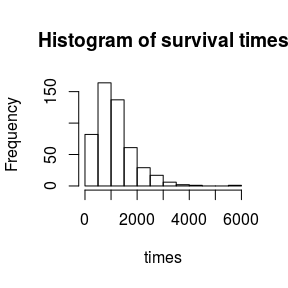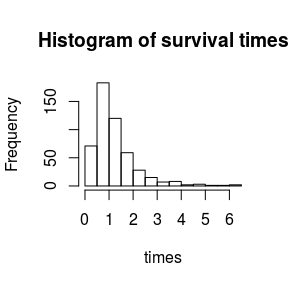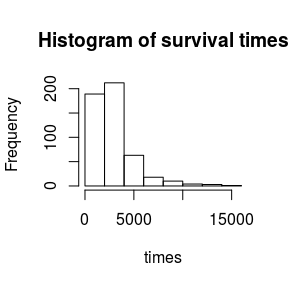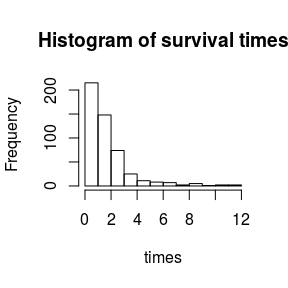# How to simulate datasets from different survival models

library(survMS)
#> Loading required package: ggplot2

# 1 Introduction

This package enables us to simulate survival data with several levels of complexity from different survival models: Cox model (Cox 1972), the Accelerated Failure Time (AFT) model, and the Accelerated Hazard (AH) model (Chen and Wang 2000). To simulate data from a Cox model, we consider the procedure of (Bender, Augustin, and Blettner 2005). This model is the most popular in survival analysis, but other models exist. The package also enables us to simulate survival data from an AFT model based on (Leemis, Shih, and Reynertson 1990). Considering different models for data simulation is interesting because the assumptions associated with these models are different. Indeed, the Cox model is a proportional risk model, not the AFT model. In the AFT model, the variables will have an accelerating or decelerating effect on on individuals’ survival. Despite the survival functions of an AFT model never intersect as in the Cox model. However, having data with intersecting survival curves allows the data to be more complex and makes it more difficult for methods to predict survival. Besides, Cox and AFT models produce data with survival curves not crossing. To have crossing survival curves, we considered two approaches. The first approach consists of modifying the AFT model to have intersecting survival curves. The second approach concerns using an AH model to generate the survival data. The AH model is more flexible than the two models mentioned above. In the AH model, the variables will accelerate or decelerate the instantaneous risk of death. The survival curves of the AH model can therefore cross each other. This package also allows us to simulate survival data from modified AFT and AH models. The generation of survival times carries out from the different models mentioned above. The models’ baseline risk function of the models is assumed to be known and follows a specific probability distribution.

## 1.1 Reminder of the functions used in survival analysis

The table below summarizes the writing of the functions used in survival analysis (instantaneous risk $$\lambda(t)$$, cumulative risk function $$H_0(t)$$, survival function $$S(t)$$ and density function $$f(t)$$) for each of the models considered (Cox, AFT and AH models).

Cox model Accelerated failure Time model Accelerated hazard model
$H(t\mid X_{i})$ $H_0(t)\exp(\beta^T X_{i})$ $H_0(t\exp(\beta^T X_{i}))$ $H_0(t\exp(\beta^T X_{i}))\exp(-\beta^T X_{i})$
$$\lambda(t\mid X_{i})$$ $\alpha_0(t)\exp(\beta^T X_{i})$ $\alpha_0(t\exp(\beta^T X_{i}))\exp(\beta^T X_{i})$ $\alpha_0(t\exp(\beta^T X_{i}))$
$$S(t\mid X_{i})$$ $S_0(t)^{\exp(\beta^T X_{i})}$ $S_0(t\exp(\beta^T X_{i}))$ $S_0(t\exp(\beta^T X_{i}))^{\exp(-\beta^T X_{i})}$
$f(t\mid X_{i})$ $f_0(t) \exp(\beta^T X_{i}) S_0(t)^{\exp(\beta^T X_{i})}$ $f_0(t\exp(\beta^T X_{i})) \exp(\beta^T X_{i})$ $f_0(t \exp(\beta^T X_{i})) S_0(t\exp(\beta^T X_{i}))^{(\exp(-\beta^T X_{i})-1)}$

## 1.2 Survival times generation

The models considered are composed of one function, $$\alpha_0(t)$$ the baseline risk and a parameter $$\beta,$$ reflecting the variables’ effect on survival times. For data generation, we assume that the baseline risk function $$\alpha_0(t)$$ is known and therefore follows a probability distribution. For this initial version of the package, the baseline hazard distributions are Weibull or log-normale. We summarize the characteristics of the distributions (Weibull/Log-normale) in the table below.

Weibull distribution Log-normal distribution
Parameters $\lambda > 0$ $a > 0$ $\mu \in ]-\infty,+\infty[$ $\sigma > 0$
Support $\mathbb{R}^+$ $\mathbb{R}^+_{\star}$
Baseline hazard $\alpha_0(t) = \lambda a t^{(a-1)}$ $\alpha_0(t) = \frac{\frac{1}{\sigma\sqrt{2\pi t}} \exp\left[-\frac{(\log t - \mu)^2 }{2 \sigma^2}\right]}{1 - \Phi\left[\frac{\log t - \mu}{\sigma}\right]}$
Cumulative Hazard $H_0(t) = \lambda t^{a}$ $H_0(t) = - \log(1 - \Phi\left[\frac{\log t - \mu}{\sigma}\right])$
Inverse cumulative hazard $H_0^{-1}(u) = \left( \frac{u}{\lambda} \right)^{1/a}$ $H_0^{-1}(u) = \exp(\sigma\Phi^{-1}(1-\exp(-u))+\mu)$
Density function $f(t) = \lambda a t^{(a-1)} \exp(-\lambda t^{a})$ $f(t) = \exp\left[-\frac{(\log t - \mu)^2 }{2 \sigma^2}\right] \frac{1}{\sigma t \sqrt{2\pi }}$
Cumulative function $F(t) = \exp(-\lambda t^{a})$ $F(t) = 1 - \Phi\left[\frac{\log t - \mu}{\sigma}\right]$
Expectation $\mathbb{E}(T) = \Gamma(\frac{1}{a} + 1) \frac{1}{\sqrt[a\,]{\lambda}}$ $\mathbb{E}(T) = \exp (\mu + \frac{\sigma^2}{2})$
Variance $\mathbb{V}(T) = \left[ \Gamma(\frac{2}{a} + 1) - \Gamma^2(\frac{1}{a} + 1)\right] \frac{1}{\sqrt[a\,]{\mu^2} }$ $\mathbb{V}(T) = (\exp(\sigma^2) -1) \exp(2\mu+\sigma^2)$

with $$\Gamma$$ is the gamma function and $$\Phi$$ is the cumulative distribution function of the standard normal distribution.

The distribution function is deduced from the survival function from the following formula:

$\begin{equation} F(t\mid X) = 1 - S(t\mid X). \end{equation}$ For data generation, if $$Y$$ is a random variable that follows a probability distribution $$F,$$ then $$U = F(Y)$$ follows a uniform distribution over the interval $$[0,1],$$ and $$(1-U)$$ also follows a uniform distribution $$\mathcal{U}[0,1].$$ We finally obtain that: \begin{align} 1 - U &= S(t\mid X) \\ %\sim \mathcal{U} [0,1] \\ &= \exp(-H_0(\psi_1(X)t)\psi_2(X)) % \sim \mathcal{U} [0,1]. \end{align} If $$\alpha_0(t)$$ is strictly positive for any $$t,$$ then $$H_0(t)$$ can be inverted and the survival time of each of the considered models (Cox, AFT, and AH) expresses for $$H_0^{-1}(u)$$. The expression of the survival times for each of the models writes in a general way: $\begin{equation} T = \frac{1}{\psi_1(X)} H^{-1}_0 \left( \frac{\log(1-U)}{\psi_2(X)} \right), \text{with} \end{equation}$ $\begin{equation} (\psi_1(X), \psi_2(X)) = \left\{ \begin{array}{ll} (1, \exp(\beta^TX)) & \mbox{for the Cox model } \\ (\exp(\beta^TX), \exp(-\beta^TX)) & \mbox{for the AH model} \\\ (\exp(\beta^TX), 1) & \mbox{for the AFT model. } \end{array} \right. \end{equation}$

Two distributions are proposed for the cumulative risk function $$H_0(t)$$ to generate the survival data. If the survival times are distributed according to a Weibull distribution $$\mathcal{W}(a, \lambda),$$ the baseline risk is of the form:

$\begin{equation} \alpha_0(t) = a\lambda t^{a-1}, \lambda > 0, a > 0. \end{equation}$ The cumulative risk function is therefore written as follows: $\begin{equation} H_0(t) = \lambda t^{a}, \lambda > 0, a > 0 \end{equation}$ and the inverse of this function is expressed as follows: $\begin{equation} H_0^{-1}(u) = \left( \frac{u}{\lambda} \right)^{1/a}. \end{equation}$ In a second step, we considered that the survival times followed a log-normal $$\mathcal{LN}(\mu, \sigma)$$ distribution of mean $$\mu$$ and standard deviation $$\sigma$$. The basic risk function is therefore written as: $\begin{equation} \alpha_0(t) = \frac{\frac{\frac{1}{\sigma\sqrt{2\pi t}}} \exp\left[-\frac{(\log t - \mu)^2 }{2 \sigma^2}\right]}{1 - \Phi\left[\frac{\log t - \mu}{\sigma}\right]}, \end{equation}$ with $$\Phi(t)$$ the distribution function of a centered and reduced normal distribution. The cumulative risk function is written as: $\begin{equation} H_0(t) = - \log\left [1 - \Phi\left(\frac{\log t - \mu}{\sigma}\right)\right] \end{equation}$ and therefore the inverse of this function is expressed by: $\begin{equation} H_0^{-1}(u) = \exp(\sigma\Phi^{-1}(1-\exp(-u))+\mu), \end{equation}$

with $$\Phi^{-1}(t)$$ the inverse of the distribution function of a centered and reduced normal distribution.

# 2 Simulation from a Cox model

We used (Bender, Augustin, and Blettner 2005) to simulate the data from a Cox model. We have chosen to carry out this simulation to generate survival data that respects the proportional risk hypothesis. We generate survival data from a Cox model as follows: $\begin{equation} T = H_0^{-1} \left[ \frac{-\log(1-U)}{\exp(\beta^T X_{i})}\right], \end{equation}$

where $$U \sim \mathcal{U} [0,1].$$

## 2.1 Simulation from a Cox model with baseline hazard following Weibull distribution

For this simulation, we consider that survival times follow a Weibull’s distribution $$\mathcal{W}(a,\lambda).$$ In this case, we have the cumulative risk function expressed by: $\begin{equation} H_0(t) = - \log\left [1 - \Phi\left(\frac{\log t - \mu}{\sigma}\right)\right] \end{equation}$ and survival times can therefore be simulated from: $\begin{equation} T = \frac{1}{\lambda^{1/a}} \left( \frac{-\log(1-U)}{\exp(\beta^T X_{i})} \right)^{1/a} . \end{equation}$
res_paramW = get_param_weib(med = 1062, mu = 1134)#compute_param_weibull(a_list = a_list, med = 2280, moy = 2325, var = 1619996)
listCoxSim_n500_p1000 <- modelSim(model = "cox", matDistr = "unif", matParam = c(-1,1), n = 500, p = 1000, pnonull = 20, betaDistr = 1,
hazDistr = "weibull", hazParams = c(res_paramW$a, res_paramW$lambda), seed = 1, d = 0)
#> Warning in modelSim(model = "cox", matDistr = "unif", matParam = c(-1, 1), :
#> Options "non-linearity with interactions" not available
#>  1.969765e+00 7.586963e-07
hist(listCoxSim_n500_p1000)## 2.2 Simulation from a Cox model with baseline hazard following log-normale distribution

For this simulation, we consider that survival times follow a log-normal distribution $$\mathcal{LN}(a,\lambda).$$ In this case, we have the cumulative risk function expressed by: $\begin{equation} H_0(t) = - \log\left [1 - \Phi\left(\frac{\log t - \mu}{\sigma}\right)\right] \end{equation}$ and survival times can therefore be simulated from: $\begin{equation} T = \exp\left(\sigma\Phi^{-1}(1-\exp\left(\frac{\log(1-U)}{\exp(\beta^TX_{i})}\right))+\mu\right). \end{equation}$

# 3 Simulation from the AFT model

To simulate the data from the AFT/Log-normal model, we used the procedure detailed in (Leemis, Shih, and Reynertson 1990). We have chosen to perform this simulation in order to generate survival data that do not respect the proportional risk hypothesis. We generate the survival data from an AFT model as: $\begin{equation} T = \frac{H_0^{-1} \left[ -\log(1-U)\right]}{\exp(\beta^T X_{i})}, \end{equation}$

where $$U \sim \mathcal{U} [0,1].$$

## 3.1 Simulation from the AFT model with baseline hazard following Weibull distribution

For this simulation, we consider that survival times follow a log-normal distribution $$\mathcal{W}(a,\lambda).$$ In this case, we have the cumulative risk function expressed by: $\begin{equation} H_0(t) = \lambda t^{a}. \end{equation}$ Survival times can therefore be simulated from: $\begin{equation} T = \left( \frac{-\log(1-U)}{\lambda}\right)^{\frac{1}{a}} \exp(-\beta^T X_{i}). \end{equation}$

## 3.2 Simulation from the AFT model with baseline hazard following log-normale distribution

For this simulation, we consider that survival times follow a log-normal distribution $$\mathcal{LN}(\mu,\sigma).$$ In this case, we have the cumulative risk function expressed by: $\begin{equation} H_0(t) = - \log\left [1 - \Phi\left(\frac{\log t - \mu}{\sigma}\right)\right], \end{equation}$ where $$\Phi(t)$$ is the distribution function of the centred and reduced normal distribution. Survival times can therefore be simulated from: $\begin{equation} T = \frac{1}{\exp(\beta^T X_{i})} \exp(\sigma \phi^{-1}(U) + \mu). \end{equation}$
listAFTSim_n500_p1000 <- modelSim(model = "AFT", matDistr = "unif", matParam = c(-1,1), n = 500, p = 100, pnonull = 100,
betaDistr = 1, hazDistr = "log-normal", hazParams = c(0.25, 0), Phi = 0, seed = 1, d = 0)
#> Warning in modelSim(model = "AFT", matDistr = "unif", matParam = c(-1, 1), :
#> Options "non-linearity with interactions" not available
hist(listAFTSim_n500_p1000)# 4 Simulation from the shifted AFT model

As mentioned above, we simulated survival data from a modified AFT model to obtain intersecting survival curves. We rewrite the cumulative risk function by adding a term $$\phi_2(X)$$: $\begin{equation} H(t|X) = H_0(t\exp(\beta^T X_{i}) + \phi_2(X)), \end{equation}$ with $$\phi_2(X) = -\beta_2^T X_{i}.$$ We get: $\begin{equation} 1 - U = \exp(-H_0(t\exp(\beta^T X_{i})) - \beta_2^T X_{i}) \sim \mathcal{U} [0,1] \end{equation}$ because $$U \sim \mathcal{U} [0,1].$$ If $$\alpha_0(t)$$ is strictly positive for any $$t,$$ then $$H_0(t)$$ can be inverted and the survival time can be expressed from $$H_0^{-1}(u)$$: $\begin{equation} T = \frac{1}{\exp(\beta^T X_{i})} \left [H^{-1}_0 \left(-\log(1-U) \right) +\beta_2^T X_{i} \right]. \end{equation}$

## 4.1 Simulation from the shifted AFT model with baseline hazard following Weibull distribution

Moreover, we suppose that the survival times follow a Weibull distribution and the inverse of the cumulative risk function is then written $$H_0^{-1}(u) = \left(\frac{u}{\lambda}\right)^{\frac{1}{a}}$$. We generate thus the survival times as follows: $\begin{equation} T = \left( \left( \frac{-\log(1-U)}{\lambda} \right)^{\frac{1}{a}} + \beta_2^T X_{i} \right) \exp(-\beta^T X_{i}). \end{equation}$

with $$U \sim \mathcal{U} [0,1].$$

## 4.2 Simulation from the shifted AFT model with baseline hazard following log-normale distribution

Moreover, we suppose that the survival times follow a log-normal distribution and the inverse of the cumulative risk function is then written $$H_0^{-1}(u) = \exp(\sigma\Phi^{-1}(1-\exp(-u))+\mu)$$ with $$\Phi^{-1}(t)$$ the inverse of the distribution function of a centered and reduced normal distribution. We generate thus the survival times as follows: $\begin{equation} T = \frac{1}{\exp(\beta^T X_{i})} \left( \exp(\sigma \Phi^{-1}(U) + \mu) + \beta_2^T X_{i} \right), \end{equation}$

with $$U \sim \mathcal{U} [0,1].$$

# res_paramLN = get_param_ln(var = 600000, mu = 1134)#compute_param_weibull(a_list = a_list, med = 2280, moy = 2325, var = 1619996)
listAFTsSim_n500_p1000 <- modelSim(model = "AFTshift", matDistr = "unif", matParam = c(-1,1), n = 500,
p = 100, pnonull = 100, betaDistr = "unif", hazDistr = "log-normal",
hazParams = c(0.2, 7.8), seed = 1, d = 0)
#> Warning in modelSim(model = "AFTshift", matDistr = "unif", matParam = c(-1, :
#> Options "non-linearity with interactions" not available
#> Warning in log(mat_grille_ti * (1/as.vector(eta_i)) - matrix(phi2, nrow(Z), :
#> production de NaN
hist(listAFTsSim_n500_p1000)# 5 Simulation from a AH model

Building on the work of (Bender, Augustin, and Blettner 2005, Leemis, Shih, and Reynertson (1990)), we also simulated survival data from another model, the AH model. We performed this simulation to generate data with intersecting survival curves. We generate the survival data from an AH model as: $\begin{equation} T = \frac{1}{\exp(\beta^T X_{i})} H_0^{-1} \left[ -\frac{\log(1-U)}{\exp(-\beta^T X_{i})}\right], \end{equation}$

with $$U \sim \mathcal{U}([0,1])$$

## 5.1 Simulation from the AH model with baseline hazard following Weibull distribution

For this simulation, we consider that survival times follow a log-normal distribution $$\mathcal{W}(a,\lambda).$$ In this case, we have the cumulative risk function expressed by: $\begin{equation} H_0(t) = \lambda t^{a}, \end{equation}$ where $$\Phi(t)$$ is the distribution function of the centred and reduced normal distribution. Survival times can therefore be simulated from: $\begin{equation} T = \left( \frac{-\log(1-U) \exp(\beta^T X_{i})}{\lambda}\right)^{\frac{1}{a}} \exp(-\beta^T X_{i}). \end{equation}$

## 5.2 Simulation from the AH model with baseline hazard following log-normale distribution

For this simulation, we consider that survival times follow a log-normal distribution $$\mathcal{LN}(\mu,\sigma).$$ In this case, we have the cumulative risk function expressed by: $\begin{equation} H_0(t) = - \log\left [1 - \Phi\left(\frac{\log t - \mu}{\sigma}\right)\right], \end{equation}$ where $$\Phi(t)$$ is the distribution function of the centred and reduced normal distribution. Survival times can therefore be simulated from: $\begin{equation} T = \frac{1}{\exp(\beta^T X_{i})} \exp\left[\sigma \Phi^{-1}\left(\frac{\log(1-U)}{\exp(-\beta^T X_{i})} \right) + \mu\right] \end{equation}$

with $$\Phi^{-1}(t)$$ the inverse of the distribution function of a centered and reduced normal distribution.

res_paramLN = get_param_ln(var = 600000, mu = 1134)#compute_param_weibull(a_list = a_list, med = 2280, moy = 2325, var = 1619996)
listAHSim_n500_p1000 <- modelSim(model = "AH", matDistr = "unif", matParam = c(-1,1), n = 500, p = 100,
pnonull = 100, betaDistr = 1, hazDistr = "log-normal",
hazParams = c(1, 0), seed = 1, d = 0)
#> Warning in modelSim(model = "AH", matDistr = "unif", matParam = c(-1, 1), :
#> Options "non-linearity with interactions" not available
hist(listAHSim_n500_p1000)# 6 Perspectives

We planned several perspectives for the package as adding interactions and non-linear effects by proposing complex functions and considering other baseline risk distributions.

## 6.1 Interactions and non-linear effects

Note that we have remained in a linear dependency framework with no interaction. The second version will take into account the non-linear and interaction framework by replacing $$\beta^T X$$ with a more complex $$g(X)$$ dependency.

## 6.2 Other distributions

We can already simulate data for the Cox model by considering Gompertz or exponential distributions for the baseline risk. The perspective is to propose also these distributions for the baseline hazard in the AFT and AH models. We wish to suggest other distributions as gamma and log-logistic distributions.